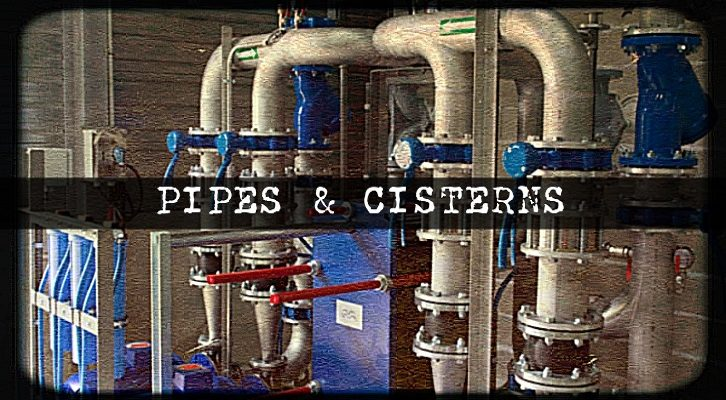# Pipes Cisterns – Questions

Have given below a few questions on pipes and cisterns.

1) Pipe A, B and C are kept open and together fill a tank in t minutes. Pipe A is kept open throughout, pipe B is kept open for the first 10 minutes and then closed. Two minutes after pipe B is closed, pipe C is opened and is kept open till the tank is full. Each pipe fills an equal share of the tank. Furthermore, it is known that if pipe A and B are kept open continuously, the tank would be filled completely in t minutes. Find t?

2) Pipe A fills a tank at the rate of 100lit/min, Pipe B fills at the rate of 25 lit/min, pipe C drains at the rate of 50 lit/min. The three pipes are kept open for one minute each, one after the other. If the capacity of the tank is 7000 liters, how long will it take to fill the tank if

i) A is kept open first, followed by B and then C
ii) B first, followed by A, and then C
iii) B first, followed by C, and then A

3) 4 men and 6 women complete a task in 24 days. If the women are at least half as efficient as the men, but not more efficient than the men, what is the range of the number of days for 6 women and 2 men to complete a task twice as difficult as this one?

4) A fill pipe can fill a tank in 20 hours, a drain pipe can drain a tank in 30 hours. If a system of n pipes (fill pipes and drain pipes put together) can fill the tank in exactly 5 hours, which of the following are possible values of n (More than one option could be correct)?
1) 32 2) 54 3) 29 4) 40